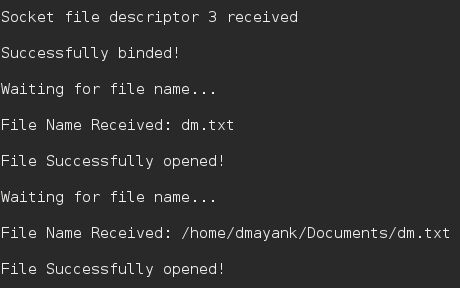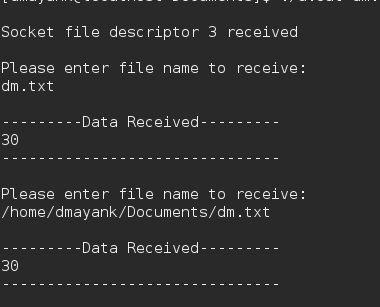# C program for file Transfer using UDP

Data can be transferred between two computers using Socket programming in C.

Similarly, files can easily be sent using UDP protocol and a simple client/server.

Security: Handled by encryption.

Protocol : UDP
Encryption: XOR encryption

Algorithm :

```1. The server starts and waits for filename.
2. The client sends a filename.
If file is present,
and continues to send a buffer filled with
file contents encrypted until file-end is reached.
4. End is marked by EOF.
5. File is received as buffers until EOF is
```

The Server :

 `// server code for UDP socket programming ` `#include ` `#include ` `#include ` `#include ` `#include ` `#include ` `#include ` `#include ` ` `  `#define IP_PROTOCOL 0 ` `#define PORT_NO 15050 ` `#define NET_BUF_SIZE 32 ` `#define cipherKey 'S' ` `#define sendrecvflag 0 ` `#define nofile "File Not Found!" ` ` `  `// function to clear buffer ` `void` `clearBuf(``char``* b) ` `{ ` `    ``int` `i; ` `    ``for` `(i = 0; i < NET_BUF_SIZE; i++) ` `        ``b[i] = ``'\0'``; ` `} ` ` `  `// function to encrypt ` `char` `Cipher(``char` `ch) ` `{ ` `    ``return` `ch ^ cipherKey; ` `} ` ` `  `// function sending file ` `int` `sendFile(``FILE``* fp, ``char``* buf, ``int` `s) ` `{ ` `    ``int` `i, len; ` `    ``if` `(fp == NULL) { ` `        ``strcpy``(buf, nofile); ` `        ``len = ``strlen``(nofile); ` `        ``buf[len] = EOF; ` `        ``for` `(i = 0; i <= len; i++) ` `            ``buf[i] = Cipher(buf[i]); ` `        ``return` `1; ` `    ``} ` ` `  `    ``char` `ch, ch2; ` `    ``for` `(i = 0; i < s; i++) { ` `        ``ch = ``fgetc``(fp); ` `        ``ch2 = Cipher(ch); ` `        ``buf[i] = ch2; ` `        ``if` `(ch == EOF) ` `            ``return` `1; ` `    ``} ` `    ``return` `0; ` `} ` ` `  `// driver code ` `int` `main() ` `{ ` `    ``int` `sockfd, nBytes; ` `    ``struct` `sockaddr_in addr_con; ` `    ``int` `addrlen = ``sizeof``(addr_con); ` `    ``addr_con.sin_family = AF_INET; ` `    ``addr_con.sin_port = htons(PORT_NO); ` `    ``addr_con.sin_addr.s_addr = INADDR_ANY; ` `    ``char` `net_buf[NET_BUF_SIZE]; ` `    ``FILE``* fp; ` ` `  `    ``// socket() ` `    ``sockfd = socket(AF_INET, SOCK_DGRAM, IP_PROTOCOL); ` ` `  `    ``if` `(sockfd < 0) ` `        ``printf``(``"\nfile descriptor not received!!\n"``); ` `    ``else` `        ``printf``(``"\nfile descriptor %d received\n"``, sockfd); ` ` `  `    ``// bind() ` `    ``if` `(bind(sockfd, (``struct` `sockaddr*)&addr_con, ``sizeof``(addr_con)) == 0) ` `        ``printf``(``"\nSuccessfully binded!\n"``); ` `    ``else` `        ``printf``(``"\nBinding Failed!\n"``); ` ` `  `    ``while` `(1) { ` `        ``printf``(``"\nWaiting for file name...\n"``); ` ` `  `        ``// receive file name ` `        ``clearBuf(net_buf); ` ` `  `        ``nBytes = recvfrom(sockfd, net_buf, ` `                          ``NET_BUF_SIZE, sendrecvflag, ` `                          ``(``struct` `sockaddr*)&addr_con, &addrlen); ` ` `  `        ``fp = ``fopen``(net_buf, ``"r"``); ` `        ``printf``(``"\nFile Name Received: %s\n"``, net_buf); ` `        ``if` `(fp == NULL) ` `            ``printf``(``"\nFile open failed!\n"``); ` `        ``else` `            ``printf``(``"\nFile Successfully opened!\n"``); ` ` `  `        ``while` `(1) { ` ` `  `            ``// process ` `            ``if` `(sendFile(fp, net_buf, NET_BUF_SIZE)) { ` `                ``sendto(sockfd, net_buf, NET_BUF_SIZE, ` `                       ``sendrecvflag,  ` `                    ``(``struct` `sockaddr*)&addr_con, addrlen); ` `                ``break``; ` `            ``} ` ` `  `            ``// send ` `            ``sendto(sockfd, net_buf, NET_BUF_SIZE, ` `                   ``sendrecvflag, ` `                ``(``struct` `sockaddr*)&addr_con, addrlen); ` `            ``clearBuf(net_buf); ` `        ``} ` `        ``if` `(fp != NULL) ` `            ``fclose``(fp); ` `    ``} ` `    ``return` `0; ` `} `

The Client:

 `// client code for UDP socket programming ` `#include ` `#include ` `#include ` `#include ` `#include ` `#include ` `#include ` `#include ` ` `  `#define IP_PROTOCOL 0 ` `#define IP_ADDRESS "127.0.0.1" // localhost ` `#define PORT_NO 15050 ` `#define NET_BUF_SIZE 32 ` `#define cipherKey 'S' ` `#define sendrecvflag 0 ` ` `  `// function to clear buffer ` `void` `clearBuf(``char``* b) ` `{ ` `    ``int` `i; ` `    ``for` `(i = 0; i < NET_BUF_SIZE; i++) ` `        ``b[i] = ``'\0'``; ` `} ` ` `  `// function for decryption ` `char` `Cipher(``char` `ch) ` `{ ` `    ``return` `ch ^ cipherKey; ` `} ` ` `  `// function to receive file ` `int` `recvFile(``char``* buf, ``int` `s) ` `{ ` `    ``int` `i; ` `    ``char` `ch; ` `    ``for` `(i = 0; i < s; i++) { ` `        ``ch = buf[i]; ` `        ``ch = Cipher(ch); ` `        ``if` `(ch == EOF) ` `            ``return` `1; ` `        ``else` `            ``printf``(``"%c"``, ch); ` `    ``} ` `    ``return` `0; ` `} ` ` `  `// driver code ` `int` `main() ` `{ ` `    ``int` `sockfd, nBytes; ` `    ``struct` `sockaddr_in addr_con; ` `    ``int` `addrlen = ``sizeof``(addr_con); ` `    ``addr_con.sin_family = AF_INET; ` `    ``addr_con.sin_port = htons(PORT_NO); ` `    ``addr_con.sin_addr.s_addr = inet_addr(IP_ADDRESS); ` `    ``char` `net_buf[NET_BUF_SIZE]; ` `    ``FILE``* fp; ` ` `  `    ``// socket() ` `    ``sockfd = socket(AF_INET, SOCK_DGRAM, ` `                    ``IP_PROTOCOL); ` ` `  `    ``if` `(sockfd < 0) ` `        ``printf``(``"\nfile descriptor not received!!\n"``); ` `    ``else` `        ``printf``(``"\nfile descriptor %d received\n"``, sockfd); ` ` `  `    ``while` `(1) { ` `        ``printf``(``"\nPlease enter file name to receive:\n"``); ` `        ``scanf``(``"%s"``, net_buf); ` `        ``sendto(sockfd, net_buf, NET_BUF_SIZE, ` `               ``sendrecvflag, (``struct` `sockaddr*)&addr_con, ` `               ``addrlen); ` ` `  `        ``printf``(``"\n---------Data Received---------\n"``); ` ` `  `        ``while` `(1) { ` `            ``// receive ` `            ``clearBuf(net_buf); ` `            ``nBytes = recvfrom(sockfd, net_buf, NET_BUF_SIZE, ` `                              ``sendrecvflag, (``struct` `sockaddr*)&addr_con, ` `                              ``&addrlen); ` ` `  `            ``// process ` `            ``if` `(recvFile(net_buf, NET_BUF_SIZE)) { ` `                ``break``; ` `            ``} ` `        ``} ` `        ``printf``(``"\n-------------------------------\n"``); ` `    ``} ` `    ``return` `0; ` `} `

Output :
Server :Client :Attention reader! Don’t stop learning now. Get hold of all the important CS Theory concepts for SDE interviews with the CS Theory Course at a student-friendly price and become industry ready.

My Personal Notes arrow_drop_upCheck out this Author's contributed articles.

If you like GeeksforGeeks and would like to contribute, you can also write an article using contribute.geeksforgeeks.org or mail your article to contribute@geeksforgeeks.org. See your article appearing on the GeeksforGeeks main page and help other Geeks.

Please Improve this article if you find anything incorrect by clicking on the "Improve Article" button below.

Improved By : shubham_singh